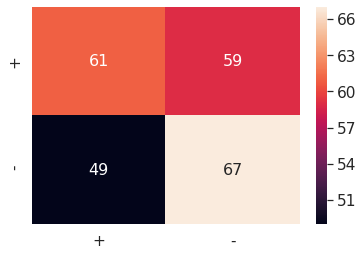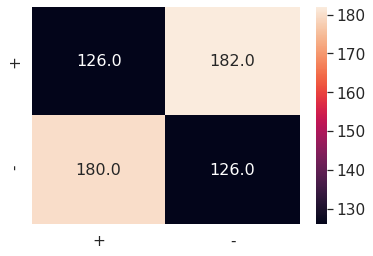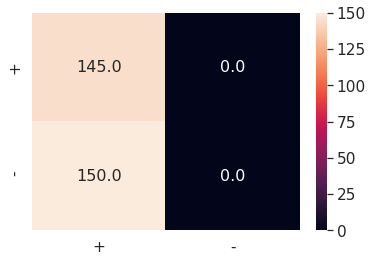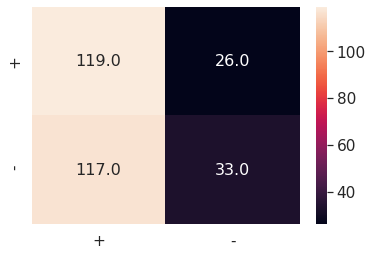PREDICTING DRUG AND ALCOHOLABUSE TREATMENT COMPLETION BY LOOKING AT GUN VIOLENCE
Share
Explore

# Models

Simple Start
To start off, I decided to try simple models that only looked at one score at a time. For these models, I simply labeled the targets as positive or negative, based on their sign. I then ran the following set of cross validators and classifiers:

cross_validators = [KFold(n_splits=K), # K = 25
ShuffleSplit(n_splits=5, test_size=.25, random_state=0),
LeaveOneOut()]

classifiers = [KNeighborsClassifier(n_neighbors=1),
KNeighborsClassifier(n_neighbors=3),
KNeighborsClassifier(n_neighbors=5),
BernoulliNB(),
SVC(kernel='linear')]
Some Results
Only a few of the results are shown below.
k-Nearest Neighbors, k = 1 || k-Fold, k = 25
Average Accuracy: 54.355%
Confusion Matrix:k-Nearest Neighbors, k =3 || Leave-One-Out
Average Accuracy: 53.390%
Confusion Matrix:Bernoulli Naive Bayes || Shuffle Split
Average Accuracy: 49.152%
Confusion Matrix:SVM, linear || Shuffle Split
Average Accuracy: 51.525%
Confusion Matrix: